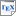[AKMMR+19] B. Aminof, M. Kwiatkowska, B. Maubert, A. Murano and S. Rubin. Probabilistic Strategy Logic. In Proc. International Joint Conference on Artificial Intelligence (IJCAI-19). To appear. 2019. [pdf] [bib] Downloads:pdf (290 KB)bib Links: [Google] [Google Scholar] [CiteSeer] Abstract. We introduce Probabilistic Strategy Logic, an extension of Strategy Logic for stochastic systems. The logic has probabilistic terms that allow it to express many standard solution concepts, such as Nash equilibria in randomised strategies, as well as constraints on probabilities, such as independence. We study the model-checking problem for agents with perfect- and imperfect-recall. The former is undecidable, while the latter is decidable in space exponential in the system and triple-exponential in the formula. We identify a natural fragment of the logic, in which every temporal operator is immediately preceded by a probabilistic operator, and show that it is decidable in space exponential in the system and the formula, and double-exponential in the nesting depth of the probabilistic terms. Taking a fixed nesting depth, this gives a fragment that still captures many standard solution concepts, and is decidable in exponential space.